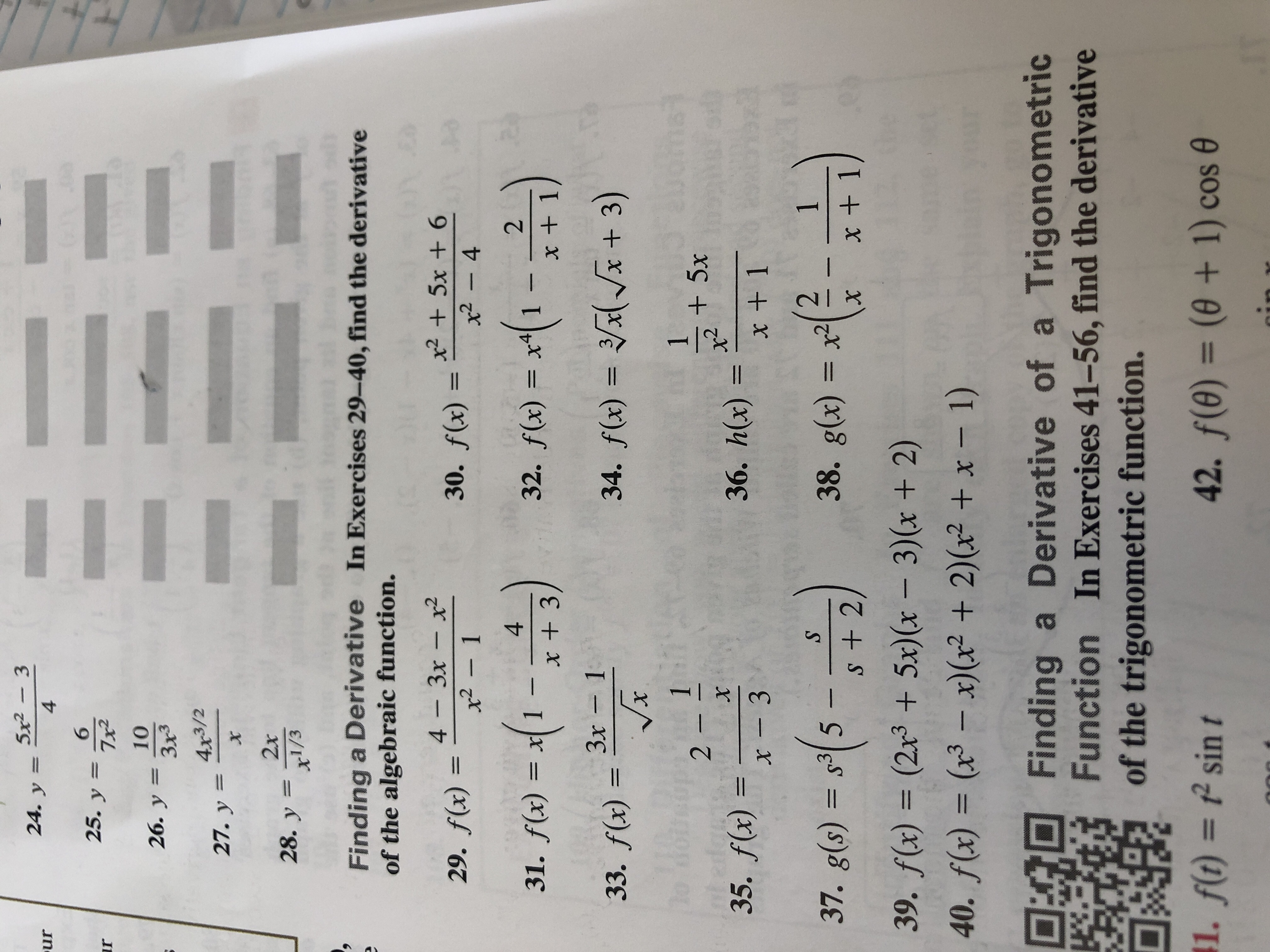# 5x2-324. y=ur4GRE625. y7x2rIo1026. y 3x3Зx34x3/227. у 3x2x28. y 1/3Finding a Derivative In Exercises 29-40, find the derivativeof the algebraic function.x25x + 6x2- 44 - Зх - х230. f(x)AS29. f(x)x2-1-(-))2431. f(x)= x32. f(x) =x + 1x + 3Зх - 1x + 3)34. f(x) =33. f(x)x12 -+5xX36. h(x)35. f(x) =x + 1x - 3138. g(x) = x237. g(s) s35 -S + 2х39. f(x) = (2x3 + 5x)(x - 3)(x + 2)40. f(x) = (x3-x)(r2 + 2)(x2 +x - 1)Finding a Derivative of a TrigonometricFunction In Exercises 41-56, find the derivativeof the trigonometric function.42. f(e)= (0 + 1) cos1. f(t)sin t44/-12

Question
1 views

31help_outlineImage Transcriptionclose5x2-3 24. y= ur 4 GRE 6 25. y 7x2 r Io 10 26. y 3x3 Зx3 4x3/2 27. у 3 x 2x 28. y 1/3 Finding a Derivative In Exercises 29-40, find the derivative of the algebraic function. x25x + 6 x2- 4 4 - Зх - х2 30. f(x) AS 29. f(x) x2-1 -(-) ) 2 4 31. f(x)= x 32. f(x) = x + 1 x + 3 Зх - 1 x + 3) 34. f(x) = 33. f(x) x 1 2 - +5x X 36. h(x) 35. f(x) = x + 1 x - 3 1 38. g(x) = x2 37. g(s) s35 - S + 2 х 39. f(x) = (2x3 + 5x)(x - 3)(x + 2) 40. f(x) = (x3-x)(r2 + 2)(x2 +x - 1) Finding a Derivative of a Trigonometric Function In Exercises 41-56, find the derivative of the trigonometric function. 42. f(e)= (0 + 1) cos 1. f(t) sin t 44/ -12 fullscreen
check_circle

Step 1

Given,

Step 2

On simplificatio...

### Want to see the full answer?

See Solution

#### Want to see this answer and more?

Solutions are written by subject experts who are available 24/7. Questions are typically answered within 1 hour.*

See Solution
*Response times may vary by subject and question.
Tagged in

### Calculus